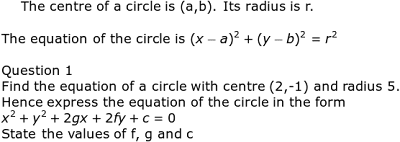## Posts

Showing posts from September, 2010

### Integration techniques Part IIIIntegrating fractions
Revision of anti chain rule "Increase power by 1 and divide by the new power"

Recall the differentiation of ln (ax + b)

### Equation of CirclesFormula for Equation of Circle